SSC BOARD PAPERS IMPORTANT TOPICS COVERED FOR BOARD EXAM 2024

### Constructions Of Triangles Class 9th Mathematics Part Ii MHB Solution

##### Constructions Of Triangles Class 9th Mathematics Part Ii MHB Solution

###### Practice Set 4.1
Question 1.

Construct Δ PQR, in which QR= 4.2cm, ∠Q = 40° and PQ+PR= 8.5cm

Given: base QR= 4.2cm, PQ+PR= 8.5cm and

∠Q = 40° of Δ PQR.

Required: To construct a ΔPQR

Steps of construction:

s

i: Draw a segment QR of length of 4.2cm.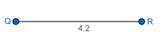ii: At vertex Q, construct ∠Q = 40° and produce a ray QB.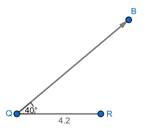iii: Mark an arc on ray QB cutting at D such that QD = 8.5cm.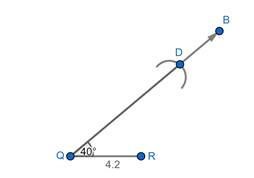iv: Draw segment DR.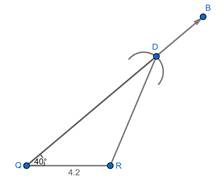v: Construct the perpendicular bisector of segment DR.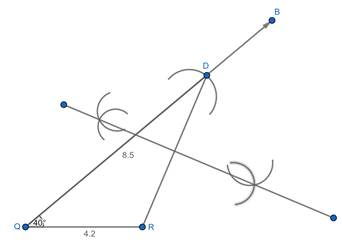vi: Name the point of intersection of ray QB and the perpendicular bisector of DR as P.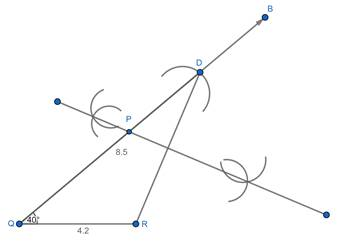vii: Draw segment RP.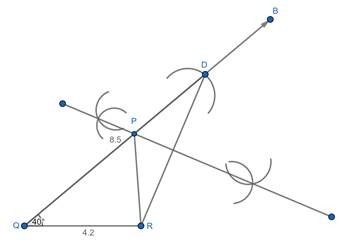ΔPQR is the required triangle.

Question 2.

Construct ΔXYZ, in which YZ= 6cm, XY+XZ= 9cm and ∠Y = 50°

Given:

base YZ= 6cm,

XY+XZ= 9cm and

∠Y = 50°

Required: To construct a ΔXYZ

Steps of construction:

i: Draw a segment YZ of length of 6cm.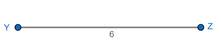ii: At vertex Y, construct ∠Y = 50° and produce a ray YA.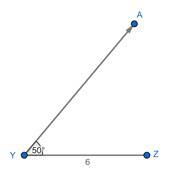iii: Mark an arc on ray YA cutting at D such that YD = 9cm.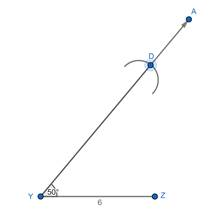iv: Draw segment ZD.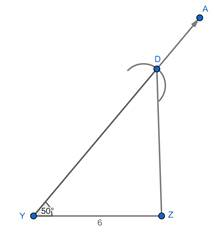v: Construct the perpendicular bisector of segment ZD.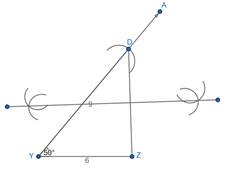vi: Name the point of intersection of ray YD and the perpendicular bisector of ZD as X.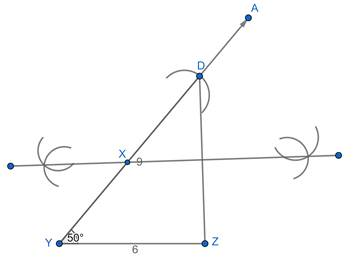vii: Draw segment XZ.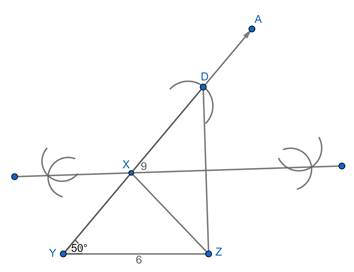ΔXYZ is the required triangle.

Question 3.

Construct Δ ABC, in which BC= 6.2cm, ∠C = 50°, AB+AC= 9.8cm

Given: base BC= 6.2cm,

AB+AC= 9.8cm and

∠C = 50° of Δ ABC.

Required: To construct a ΔABC

Steps of construction:

i: Draw a segment BC of length of 6.2cm.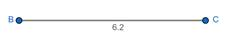ii: At vertex C, construct ∠C = 50° and produce a ray CP.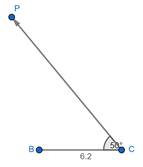iii: Mark an arc on ray CP cutting at D such that CD = 9.8cm.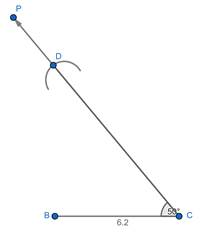iv: Draw segment DB.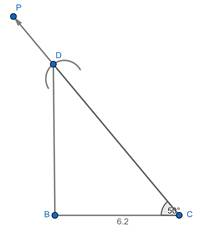v: Construct the perpendicular bisector of segment DB.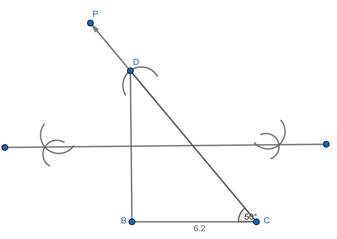vi: Name the point of intersection of ray CP and the perpendicular bisector of DB as A.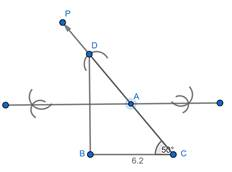vii: Draw segment AB.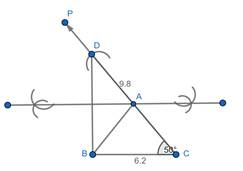ΔABC is the required triangle.

Question 4.

Construct ΔABC, in which BC= 5.2cm, ∠C = 45° and perimeter of ΔABC is 10 cm.

Given: base BC= 5.2cm, ∠C = 45° of ΔABC and

perimeter of ΔABC = 10 cm.

Required: To construct a ΔABC

Steps of construction:

i: Draw a segment BC of length of 5.2cm.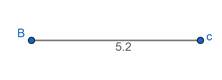ii: At vertex C, construct ∠C = 45° and produce a ray P’C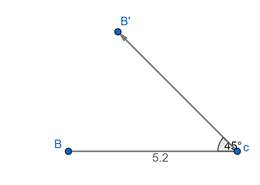iii: Mark an arc on ray CP cutting at D such that CD = 4.8cm.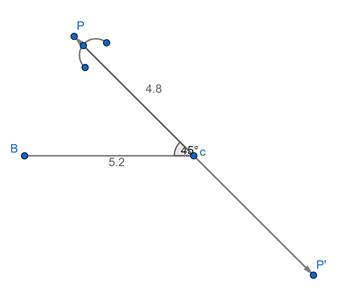iv: Draw segment DB.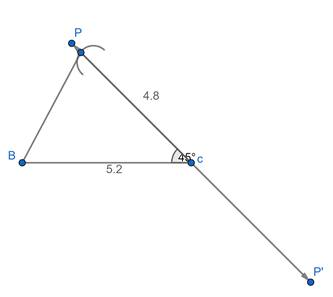v: Construct the perpendicular bisector of segment DB.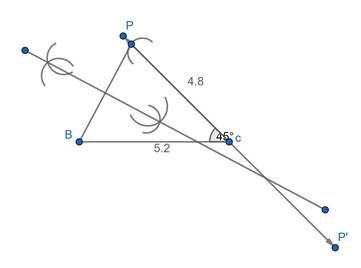vi: Name the point of intersection of ray CP and the perpendicular bisector of DB as A.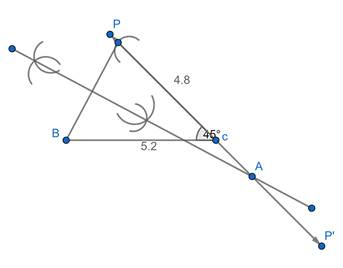vii: Draw segment AB.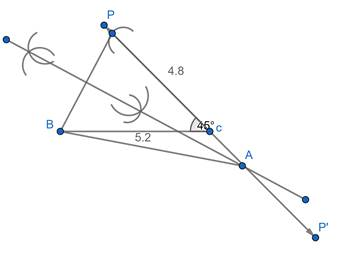ΔABC is the required triangle.

###### Practice Set 4.2
Question 1.

Construct Δ XYZ, in which YZ= 7.4cm, ∠XYZ = 45° and XY-XZ= 2.7cm

Given: Base YZ= 7.4cm, XY-XZ= 2.7cm and

∠Y = 45° of Δ XYZ.

Required: To construct a triangle XYZ.

Steps of construction:

i: Draw a segment YZ of length 7.4cm.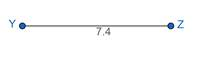ii: Draw ray YL such that ∠Y = 45°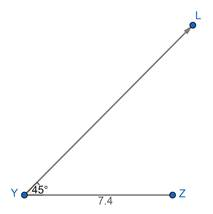iii: Mark an arc on ray YL cutting at D such that YD = 2.7cm.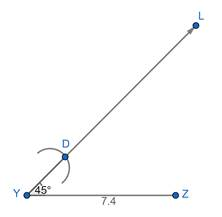iv: Draw segment ZD.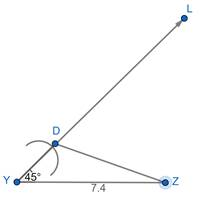v: Construct the perpendicular bisector of segment ZD.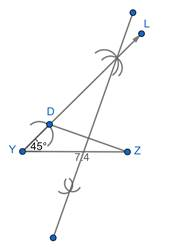vi: Name the point of intersection of ray YL and the perpendicular bisector of ZD as X.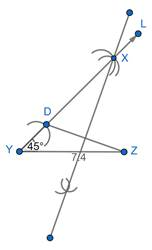vii: Draw segment XZ.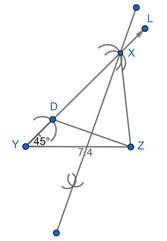ΔXYZ is the required triangle.

Question 2.

Construct Δ PQR, in which QR = 6.5cm, ∠PQR = 60° and PQ-PR = 2.5cm

Given: Base QR = 6.5cm,

PQ-PR = 2.5cm and

∠Q = 60° of Δ PQR.

Required: To construct a triangle PQR.

Steps of construction:

i: Draw a segment QR of length 6.5cm.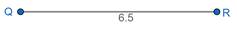ii: Draw ray QL such that ∠Q = 60°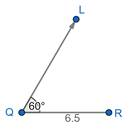iii: Mark an arc on opposite ray QL i.e. QS cutting at D such that QD = 2.5cm.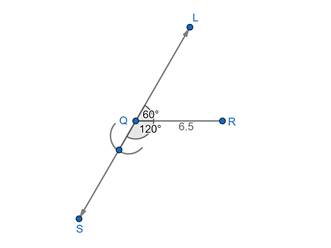iv: Draw segment RD.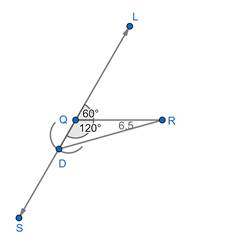v: Construct the perpendicular bisector of segment RD.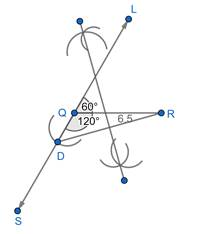vi: Name the point of intersection of ray QL and the perpendicular bisector of RD as P.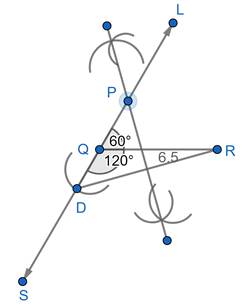vii: Draw segment PR.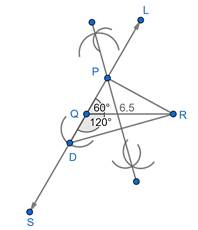ΔPQR is the required triangle.

Question 3.

Construct Δ ABC, in which BC = 6cm, ∠ABC = 100° and AC – AB – 2.5CM

Given: Base BC = 6cm, AC-AB = 2.5cm and

∠B = 100° of Δ ABC.

Required: To construct a triangle ABC.

Steps of construction:

i: Draw a segment BC of length 6cm.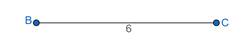ii: Draw ray BT such that ∠B = 100°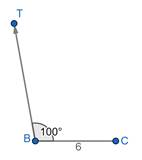iii: Mark an arc on opposite of ray BT i.e. ray BS cutting at D such that BD = 2.5cm.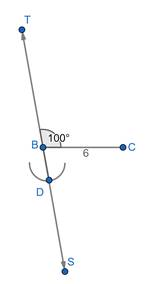iv: Draw segment CD.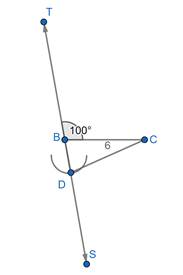v: Construct the perpendicular bisector of segment CD.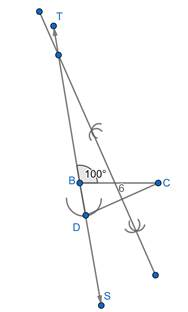vi: Name the point of intersection of ray BS and the perpendicular bisector of CD as A.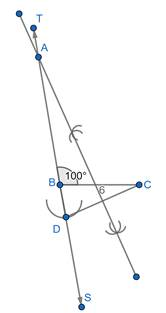vii: Draw segment AC.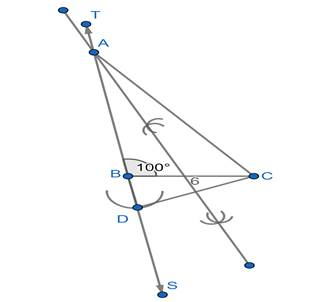ΔABC is the required triangle.

###### Practice Set 4.3
Question 1.

Construct Δ PQR, in which ∠Q =70°, ∠R = 80° and PQ+QR+PR=9.5cm

Given: ∠Q =70°,

∠R = 80° and

perimeter of Δ PQR

Steps of construction:

i: Draw a line segment XY of 9.5cm.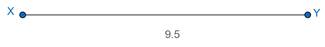ii: From point X draw a ray XD at 70° and from Y draw a ray YE at 80°.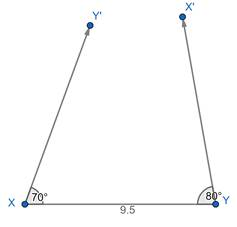iii: Draw an angle bisector of X and Y, two angle bisectors intersect each other at point A.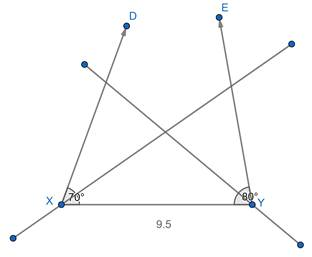iv: Draw a line bisector of XA and AY respectively these two-line bisectors intersect at point B and C.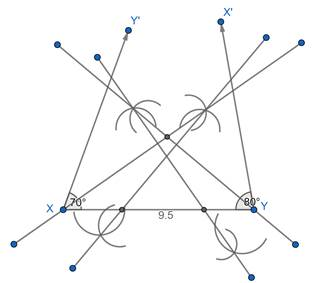v: Join AB and AC.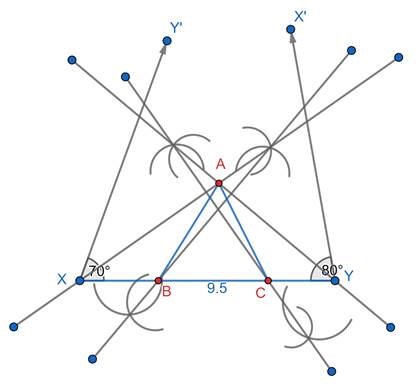vi: Δ ABC is required triangle.

Question 2.

Construct ΔXYZ in which ∠Y =58°, ∠X = 46° and perimeter of triangle is 10.5 cm.

Given: ∠Y =58°, ∠X = 46° and perimeter of Δ PQR

Steps of construction:

i: Draw a line segment QR of 10.5cm.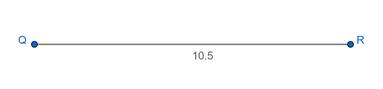ii: From point Q draw a ray QD at 58° and from R draw a ray RE at 46°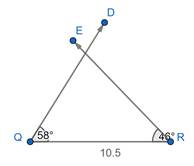iii: Draw an angle bisector of Q and R, two angle bisectors intersect each other at point X.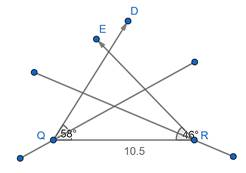iv: Draw a line bisector of QX and XR respectively these two-line bisectors intersect at point Y and Z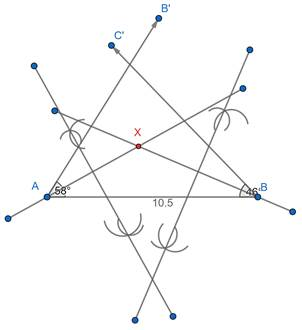v: Join XY AND XZ.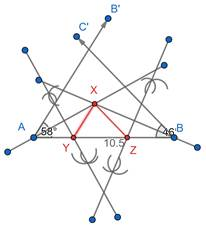vi: Δ XYZ is required triangle.

Question 3.

Construct ΔLMN, in which ∠M =60°, ∠N =80° and LM+MN+NL=11cm.

Given: ∠Q =70°,

∠R = 80° and

perimeter of Δ PQR

Steps of construction:

i: Draw a line segment AB of 11cm.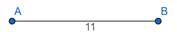ii: From point A draw a ray AD at 60° and from B draw a ray BE at 80°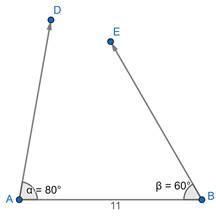iii: Draw an angle bisector of A and B, two angle bisectors intersect each other at point P.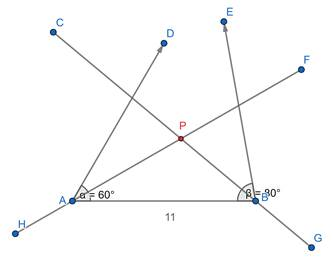iv: Draw a line bisector of AP and BP respectively these two-line bisectors intersect at point Q and R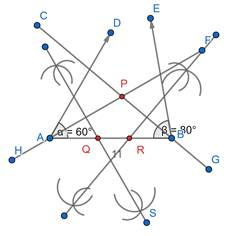v: Join PQ AND PR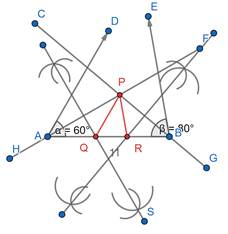vi: Δ PQR is required triangle.

###### Problem Set 4
Question 1.

Construct ΔXYZ, such that XY+XZ= 10.3cm, YZ=4.9cm, ∠XYZ = 45°

Given: base YZ= 6cm, XY+XZ= 9cm and ∠Y = 50°

of ΔXYZ.

Required: To construct a ΔXYZ

Steps of construction:

i: Draw a segment YZ of length of 4.9cm.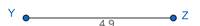ii: At vertex Y, construct ∠Y = 45° and produce a ray YA.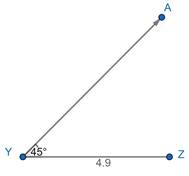iii: Mark an arc on ray YA cutting at D such that YD = 9cm.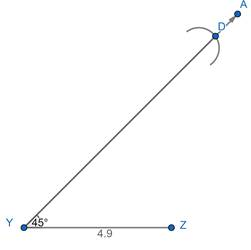iv: Draw segment ZD.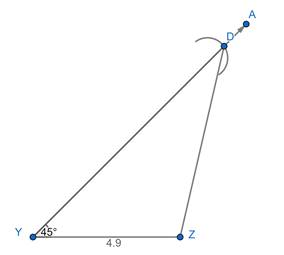v: Construct the perpendicular bisector of segment ZD.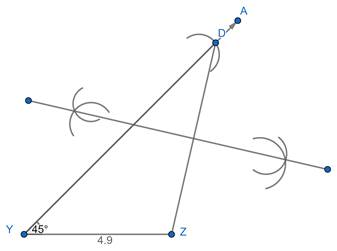vi: Name the point of intersection of ray YD and the perpendicular bisector of ZD as X.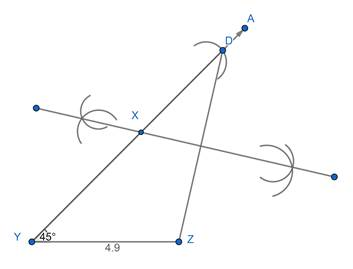vii: Draw segment XZ.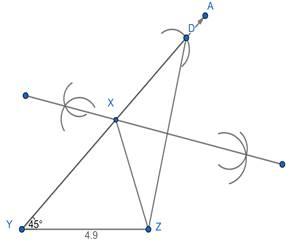ΔXYZ is the required triangle.

Question 2.

Construct Δ ABC, in which ∠B =70°, ∠C = 60°, AB+BC+AC=11.2cm.

Given: ∠B =70°, ∠C = 60° and perimeter of Δ ABC

Steps of construction:

i: Draw a line segment PQ of 11.2cm.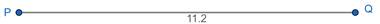ii: From point P draw a ray PD at 70° and from Q draw a ray QE at 60°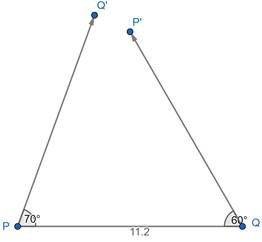iii: Draw an angle bisector of P and Q, two angle bisectors intersect each other at point A.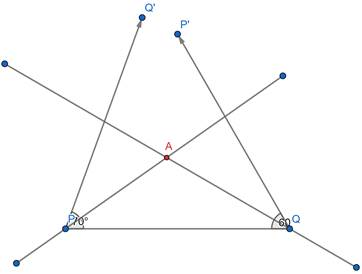iv: Draw a line bisector of AP and AQ respectively these two-line bisectors intersect at point B and C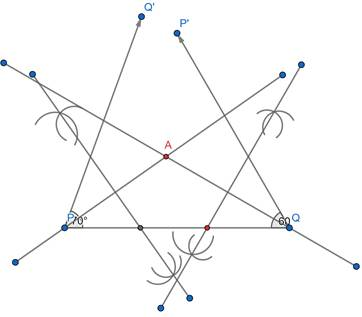v: Join AB AND AC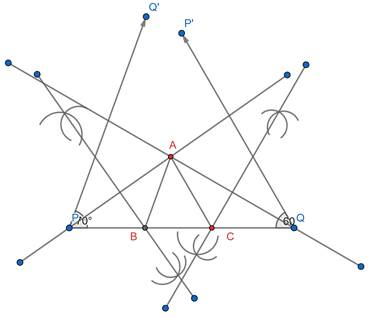vi: Δ ABC is required triangle.

Question 3.

The perimeter of a triangle is 14.4 cm and the ratio of lengths of its side is 2: 3: 4. Construct the triangle.

Given: perimeter of Δ = 14.4cm and ratio of its sides = 2:3:4

Steps of construction:

i: Draw B’C’ = 9cm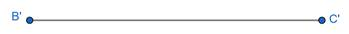ii: Then divide B’C’ in the ratio 2:3:4. B’B:BC∷CC’ = 2:3:4 (by similarity of triangles)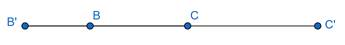iii: Now construct AB = B’B.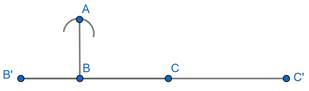iv: Construct AC = CC’.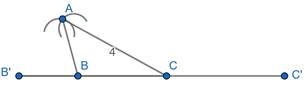v: Hence, AB:BC:AC = 2:3:4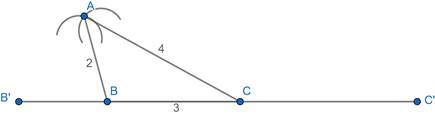vi: Hence, Δ ABC is the required triangle.

Question 4.

Construct Δ PQR, in which PQ-PR= 2.4cm, QR= 6.4cm and ∠PQR = 55°.

Given: Base QR= 6.4cm, PQ-PR= 2.4cm and

∠Q = 545° of Δ PQR.

Required: To construct a triangle PQR.

Steps of construction:

i: Draw a segment QR of length 6.4cm.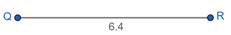ii: Draw ray QL such that ∠Q = 55°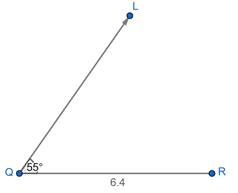iii: Mark an arc on ray QL cutting at D such that QD = 2.4cm.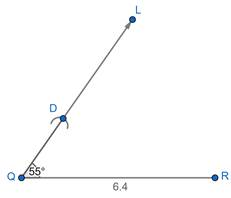iv: Draw segment RD.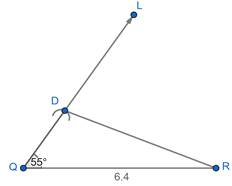v: Construct the perpendicular bisector of segment RD.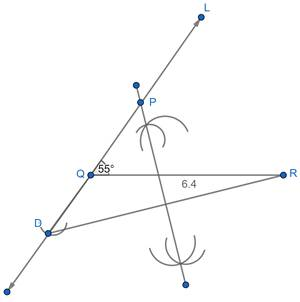vi: Name the point of intersection of ray QL and the perpendicular bisector of RD as P.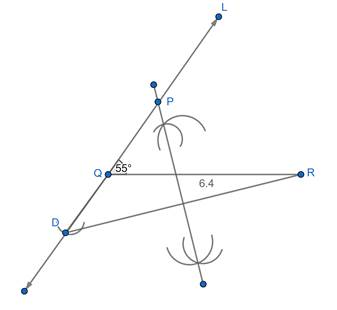vii: Draw segment PR.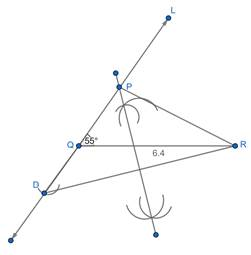ΔPQR is the required triangle.